## Thursday, July 26, 2007

### Irodov Problem 1.51The first question to ask before solving this question is "what is special about the axis of rotation of a rigid body?". The answer is that since the entire body rotates about this axis, at any instant every point in the rigid body the velocity vector and the radial vector (line connecting point to the axis in the plane of rotation) must be perpendicular to each other. This is illustrated in the figure for an arbitrarily shaped rigid body. Depicted in the figure are velocity vectors of several different points in the rigid body and the radial vectors that connect the point to the axis of rotation. For every point these will be perpendicular to each other. In other words if we draw lines perpendicular to the velocity vector at any point in the rigid body in the plane of rotation, they must all pass through the axis of rotation. We shall use this fact to solve the problem.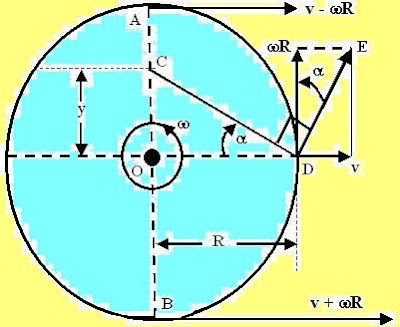In the figure we consider two points A (at the top-most point on the disc) and C (at a point where the x-axis intersects the rim of the disc) on the rim of the disc. The velocity vector of A
is oriented along the x-axis, thus the axis of rotation must lie somewhere along the line AB (the perpendicular to the velocity vector at A) which is the diameter of the circle. The velocity vector at point D is DE and the perpendicular to DE at D is DC. The axis of rotation must thus lie at C - the intersection point of the two perpendiculars. Thus, to solve this problem we need to determine y, which is the length OC.

Suppose that the radius of the disc is R. Further suppose that the current angular velocity of the disc with respect to the center is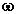. If the velocity vector DE is oriented at an anglethen,
from the figure,Also from the figure it is clear that,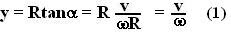Now we can use (1) to solve both parts of the question.

(a) For this part,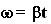. Thus, the location of the axis of rotation in Cartesian coordinates is given by,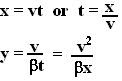(b) For this part,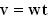. Thus, the location of the axis in this case is given by,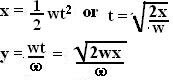Anonymous said...

You can also use the fact that the velocity of the instantaneous axis of rotation is zero with respect to the outside frame.Anonymous said...

Thanx sir.

prakash G said...

If you use the instanteneous axis of rotation has zero velocity answer will come in one line.Anonymous said...

Exactly .... using that fact makes solution 2 lines long!

Unknown said...

agree too...
thanx for the clueAnonymous said...

I must say, everythin' was very well explained